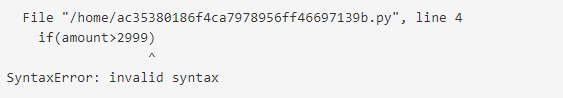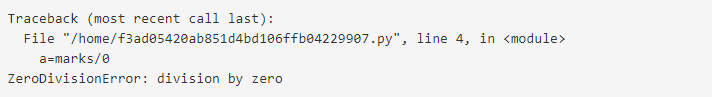# Python Exception Handling

We have explored basic python till now from Set 1 to 4 (Set 1 | Set 2 | Set 3 | Set 4).

Error in Python can be of two types i.e. Syntax errors and Exceptions. Errors are the problems in a program due to which the program will stop the execution. On the other hand, exceptions are raised when some internal events occur which changes the normal flow of the program.

## The difference between Syntax Error and Exceptions

Syntax Error: As the name suggest this error is caused by wrong syntax in the code. It leads to the termination of the program.

Example

 `# initialize the amount variable ` `amount ``=` `10000` ` `  `# check that You are eligible to ` `#  purchase Dsa Self Paced or not ` `if``(amount>``2999``) ` `    ``print``(``"You are eligible to purchase Dsa Self Paced"``) ` `    `

Output:Exceptions: Exceptions are raised when the program is syntactically correct but the code resulted in an error. This error does not stop the execution of the program, however, it changes the normal flow of the program.

Example:

 `# initialize the amount variable ` `marks ``=` `10000` ` `  `# perform division with 0 ` `a ``=` `marks ``/` `0` `print``(a) `

Output:In the above example raised the ZeroDivisionError as we are trying to divide a number by 0.

Note: Exception is the base class for all the exceptions in Python. You can check the exception hierarchy here.

## Try and Except in Exception Handling

Let us try to access the array element whose index is out of bound and handle the corresponding exception.

 `# Python program to handle simple runtime error ` ` `  `a ``=` `[``1``, ``2``, ``3``] ` `try``:  ` `    ``print` `"Second element = %d"` `%``(a[``1``]) ` ` `  `    ``# Throws error since there are only 3 elements in array ` `    ``print` `"Fourth element = %d"` `%``(a[``3``])  ` ` `  `except` `IndexError: ` `    ``print` `"An error occurred"`

Output:

```Second element = 2
An error occurred
```

A try statement can have more than one except clause, to specify handlers for different exceptions. Please note that at most one handler will be executed.

 `# Program to handle multiple errors with one except statement ` `try` `:  ` `    ``a ``=` `3` `    ``if` `a < ``4` `: ` ` `  `        ``# throws ZeroDivisionError for a = 3  ` `        ``b ``=` `a``/``(a``-``3``) ` `     `  `    ``# throws NameError if a >= 4 ` `    ``print` `"Value of b = "``, b ` ` `  `# note that braces () are necessary here for multiple exceptions ` `except``(ZeroDivisionError, NameError): ` `    ``print` `"\nError Occurred and Handled"`

Output:

`Error Occurred and Handled`

If you change the value of ‘a’ to greater than or equal to 4, the output will be

```Value of b =
Error Occurred and Handled
```

The output above is so because as soon as python tries to access the value of b, NameError occurs.

### Else Clause

In python, you can also use else clause on the `try-except` block which must be present after all the except clauses. The code enters the else block only if the try clause does not raise an exception.

 `# Program to depict else clause with try-except ` ` `  `# Function which returns a/b ` `def` `AbyB(a , b): ` `    ``try``: ` `        ``c ``=` `((a``+``b) ``/` `(a``-``b)) ` `    ``except` `ZeroDivisionError: ` `        ``print` `"a/b result in 0"` `    ``else``: ` `        ``print` `c ` ` `  `# Driver program to test above function ` `AbyB(``2.0``, ``3.0``) ` `AbyB(``3.0``, ``3.0``) `

The output for above program will be :

```-5.0
a/b result in 0
```

### Finally Keyword in Python

Python provides a keyword finally, which is always executed after try and except blocks. The finally block always executes after normal termination of try block or after try block terminates due to some exception.

Syntax:

```try:
# Some Code....

except:
# optional block
# Handling of exception (if required)

else:
# execute if no exception

finally:
# Some code .....(always executed)
```

Example:

 `# Python program to demonstrate finally  ` `   `  `# No exception Exception raised in try block  ` `try``:  ` `    ``k ``=` `5``/``/``0` `# raises divide by zero exception.  ` `    ``print``(k)  ` `   `  `# handles zerodivision exception      ` `except` `ZeroDivisionError:     ` `    ``print``(``"Can't divide by zero"``)  ` `       `  `finally``:  ` `    ``# this block is always executed   ` `    ``# regardless of exception generation.  ` `    ``print``(``'This is always executed'``)   `

Output:

```Can't divide by zero
This is always executed
```

## Raising Exception

The raise statement allows the programmer to force a specific exception to occur. The sole argument in raise indicates the exception to be raised. This must be either an exception instance or an exception class (a class that derives from Exception).

 `# Program to depict Raising Exception ` ` `  `try``:  ` `    ``raise` `NameError(``"Hi there"``)  ``# Raise Error ` `except` `NameError: ` `    ``print` `"An exception"` `    ``raise`  `# To determine whether the exception was raised or not `

The output of the above code will simply line printed as “An exception” but a Runtime error will also occur in the last due to raise statement in the last line. So, the output on your command line will look like

```Traceback (most recent call last):
File "003dff3d748c75816b7f849be98b91b8.py", line 4, in
raise NameError("Hi there") # Raise Error
NameError: Hi there
```